## Articulo

•Citado por SciELO
•Accesos

•Similares en SciELO

## versión impresa ISSN 0583-7693

### Rev. Soc. Quím. Méx vol.46 no.4 Ciudad de México oct./dic. 2002

Investigación

Ammonium Azide: A Commented Example of an Ab Initio Structure (Re-)Determination From X-Ray Powder Diffraction

Helio Salim de Amorim,1* M. Rothier do Amaral Jr.,1 Philip Pattison,2 Isabel Pereira Ludka3 and Julio Cesar Mendes3

1 Instituto de Física, Universidade Federal do Rio de Janeiro, CP 68528, 21945-970, Rio de Janeiro-RJ; Phone: (55)(21) 2562-7372; Fax: (55)(21) 2562-7368. E-mail: hsalim@if.ufrj.br

2 Institute of Crystallography, BSP, University of Lausanne, CH-1015, Lausanne-Switzerland.

3 Departamento de Geología, Universidade Federal do Rio de Janeiro, 21949-900 Rio de Janeiro-RJ.

Recibido el 7 de junio del 2002.
Aceptado el 21 de noviembre del 2002.

Abstract

The structure of ammonium azide (NH4)N3 was (re-)deter-minated ab initio from x-ray powder diffraction experiment using synchrotron radiation. We tried to detail and comment the different steps involved in the structure determination. The compound crysta-llize in the orthorhombic Pmna space group (no.53) with a = 8.937(1) Å, b = 3.8070(5) Å, c = 8.664(1) Å, V = 294.79(7) Å3; Z = 4. It was possible to locate and refine the hydrogen coordinates, in two diffe-rent approaches, and to establish the H-bonds. The final structural parameters are in good agreement with previous results based on three-dimensional neutron diffraction.

Key words: Ammonium azide; x-ray diffraction; structure determination.

Resumen

La estructura de la azida de amonio (NH4)N3 fue redeterminada mediante ab-initio a partir de los datos de difracción de rayos X empleando eadiación de sincrotrón. El compuesto cristaliza en el grupo espacial Pmna (no.53) con a = 8.937(1) Å, b = 3.8070(5) Å, c = 8.664(1) Å, V = 294.79(7) Å3; Z = 4. Fue posible localizar y refinar las coordenadas de hidrógeno, en dos aproximaciones diferentes, y establecer las uniones de hidrógeno. Los parámetros finales de la estructura están en buena concordancia con resultados previos basados en el análisis de la difracción tridimensional de neutrones.

Palabras clave: Azida de amonio, difracción de rayos X, determinación estructural.

Introduction

In the last few years, many new methods for the determination of crystal structures by x-ray diffraction of polycrystalline samples have been developed. The increase in the number and range of methods has been stimulated by the practical needs to solve the structures of new compounds, particularly those from the pharmaceutical area. These samples often present serious difficulties to obtain single crystals of a size and per-fection suitable for analysis by conventional techniques of x-ray diffraction. On the other hand, this increase has been pos-sible thanks to the improvement in the capacity for processing experimental data and to the significant technical improvements of the new x-ray powder diffraction (XRPD) equipment. In this last aspect, the development in the use of syn-chrotron light sources has been very significant. The structure of various relatively difficult compounds has been reported based on these advances, such as the uranyl phosphonate (UO2)3(HO3PC6H5)2.H2O with 50 non-H atoms  and La3Ti5 Al15O37 with 60 atoms  in the asymmetric unity.

The basic problem of the powder method, as applied to the determination of crystal structures, is that the experimental data correspond to a sort of projection, in only one dimension, of all contributions of the three-dimensional reciprocal lattice. A diffractogram is typically a discrete set of intensity data (I) of the radiation scattered by the polycrystalline sample versus the scattering angle (2θ). The maxima of constructive interference or Bragg lines, which contain information about the structure that one wants to determine, are hardly ever found isolated. All the Bragg lines with identical, or very close, values of interplanar distances contribute to the same peak. This superposition may be intrinsic, due to the symmetry of crystal lattice, or connected to the line broadening that coalesce different contributions in a single line. This line broadening is due to the structural defects and instrumental factors that limit the diffractometer angular resolution. This superposition of diffraction lines ends up by preventing us in practical terms from obtaining a indexed list of isolated Bragg lines with the respective modulus of structure factors, preventing us from trying to obtain the structure solution by means of direct methods or methods based on the Patterson function.

A significant advance towards obtaining three-dimensional structural information from such projected data as a powder diffractogram arises with the Rietveld method , for the refinement of crystal structure. All the present methods to determine the structures from polycrystalline samples, as well as other uses of XRPD, have benefited from this contribution. A second significant stage of this evolution was the introduction of methods that start with an arbitrary model and apply successive changes so as to approach the calculated to the observed diffratogram. These changes in the initial structure are made by an algorithm based on the Monte Carlo method and simulated annealing approaches. These methods are called, in general, direct space methods since they manipulate the structure in the physical space (in contrast with the classi-cal methods that use properties of the reciprocal space). The fundamental advantage of these methods is that they do not require the decomposition of the diffraction pattern into sepa-rate peaks or, in other words, the extraction of structure fac-tors. A general review about the advances of XRPD tech-niques and the uses of synchrotron radiation, in particular con-cerning the determination of crystal structures, may be found in the works by Louër (1998)  and Helliwel (1998) , respectively.

The aim of this work is to evaluate the extension of the structure properties that we can obtain from a high resolution powder diffractogram to the challenging compound for the conventional XRPD technique: ammonium azide (NH4)N3. This is a compound with elements of low atomic number and it includes hydrogen as an essential element for the description of the structure. The location of hydrogen is difficult with the x-ray scattering, even for the studies with single crystals, and the use of neutron diffraction is recommended in this case. The first study with ammonium azide was made by Frevel  (1936), who determined the positions of nitrogen atoms and inferred from the N-N distances the existence of strong H-bonds. This indicates a definite orientation, at room temperature, of the (NH4)1+ ions, instead of rotating states that would result in a spherical distribution to the ion. A second study was presented by Prince and Choi , who refined the positions of the hydrogen atoms from neutron diffraction data on a single crystal, and hence completed the description of the compound. The study revealed a very regular tetrahedral con-figuration of the ion (NH4)1+.

Experimental

The powder sample of ammonium azide was prepared by the reaction of NaN3 with NH4Cl in N,N-dimethylformamide as described by Evans  et al. A finely ground sample was inserted into a 0.5 mm borate capillary on the powder diffractometer of the Swiss-Norwegian beamline (SNBL) on BM1B at the European Synchrotron Radiation Facility (ESRF). The data were collected at room temperature in the angular interval (2θ) from 5° to 47.560° in steps of 0.004°. The sample was rotated around the capillary axis throughout the measurement in order to improve the statistical averaging of the diffraction from different grains in the sample and to reduce any contribution from preferred orientation. The wavelength of 0.59716 Å was selected using a Si(111) monochromator, and calibrated before the start of the experiment using a Si powder reference sample (NBS 650B). The intrinsic angular resolution of the diffractometer is determined mainly by the Si(111) analyzer crystal in the diffracted beam, which results in an instrumental FWHM of about 0.01° in 2θ. The experimental diffraction pattern is presented in Fig. 1.Method of data analysis

In order to characterize the ammonium azide structure we used a strictly ab initio approach. In the following sections we tried to detail and comment a little more some of the practical aspects that involved the structure determination from a powder diffractogram. Our concern here is with a non-specialist in this area but that uses XRPD in its most conventional ways. We also tried to give information about all the programs that were applied.

Indexing of the diffraction pattern

Special emphasis was placed on locating the first few reflections at low angle. These reflections are generally less super-imposed, since the overlap tends to increase when the diffraction angle increases, and they form a very important set of lines for the selection of a crystallographic space group. The location of the lines is automatically made with help of programs such as ESPECTRO, based on the method of the second derivative and developed in our laboratory (Laboratório de Cristalografia e Raio-x / IF-UFRJ) but, the program FULL-PROF by Rodriguez Carvajal  achieved this aim equally well.

From the set of Bragg angles (2 θB), we carry out the indexing. At this stage it is crucial to have good starting data in which all the efforts to minimize the influence of systematic errors have been made. To proceed beyond this stage, it is important to know the density of compound. We use the value 1,352 g.cm-3 provided by Prince and Choi .

The methods for auto-indexing the diffraction pattern have been greatly improved. We have used the program developed by Taupin  that is based on attribution of Miller indexes to an initial set of lines in order to form a system of equations, relating the measured interplanar distances and the cell constants of a Bravais system chosen at first. The solution of the equations system results in parameters of a provisional cell that are used to try the indexing of the other lines. The program tests the solutions by calculating the number of mole-cules by unit cell (Z) from the volume of the cell, the mea-sured density of compound and the molecular weight, selecting those that results in a integer value for Z within the assu-med experimental errors. The acceptable solutions are selected with the calculus of a figure of merit. Among the most used figures of merit is the parameter M20 introduced by de Wolf , based on the quality of the fit between the calculated and measured interplanar distances as well as on the size of unity cell. There is not an absolute value for M20 that assures the correctness of the adopted solution, however it is generally accepted that a solution with M20 >20 is usually correct. If no acceptable solution is found a new Bravais system is adopted and the method is reinitiated.

In Table 1, we present the result of the indexing of the first 34 lines based on an orthorhombic cell that results in Z = 4. The analysis of indexes permits us to verify that it is a P-type lattice and to associate the conditions: (h0l), h + l = 2n and (hk0), h = 2n with allowed reflections. These conditions result in the symbol of diffraction mmm P n c -corresponding to two possible space groups, Pmna (no. 53) and Pnc2 (no. 30). The group Pnc2 is non-centrosymmetric, it has only two special positions and the highest point symmetry is 2. The Pmna group is centrosymmetric; it has a larger number of spe-cial positions and higher point symmetries such as 2 / m. These differences permit a definition of the space group in the following stages but, owing to the geometric characteristics of ions N31- and (NH4)1+ it is more probable that these ions are in lattices that have inversion symmetries and mirror planes. As a starting point we therefore adopted the group Pmna.Decomposition of the diffractogram

At this stage we use the Le Bail method . This method is a special application of Rietveld analysis where the aim in this case is not the refinement of the atomic coordinates, but rather to obtain the square of structure factors |Fj|2 for all reflections in the space group Pmna within the measured interval of 2θ.

The method consists of describing the diffractogram through the Rietveld equation,where Φ(2 θi - 2θj) is an analytic function, normalized, selected to describe the Bragg line profile, mj is the multiplicity factor of the reflection j and (Lp)j is the Lorentz and polarization factor. The summation is over all the neighboring Bragg lines and the function b(2 θi) describes the background intensity. The coefficients that describe the line profile and the cell para-meters are evaluated using the least-squares method. The parameters |Fj|2 are evaluated by an iterative method: starting with a complete set of Bragg reflections with equal |Fj|, a internal sequence of last-squares calculations determines new optima values to cell and profile parameters. Afterwards, a new set of |Fj|'s is calculated by direct comparison of equation (1) with the measured intensities (Imeas(2 θi)). This sequence of calculations is repeated until convergence is achieved.

Those reflections which are completely overlapped have the intensity naturally equipartitioned by the process. We assume that the sample does not present preferential orientation effects. The program that we have used to implement this method was FULLPROF. The obtained R-factors were Rwp = 0.18 (Rexpected = 0.175) and S = 1.02. The final file with the results contains 407 reflections, with 268 non-null reflections.

We tried to analyze the distribution of the values | Fj|. The result, involving the 407 reflections, can be observed in Fig. 2. The plot clearly suggest a typical distribution of a centrosym-metric structure , supporting the choice of Pmna group. The complete set of 407 reflections was then used, in the direct methods approach, for structure solution.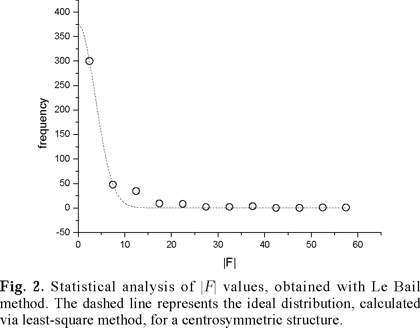Locations of non-H atoms via direct methods

The nitrogen atoms were located by direct methods with SIR97 . The results are presented in Table 2. The final residue of 0.156 indicates an adequate structural model as a starting point to refinement. We can recognize the presence of two independent azide ions, formed by N(1) in the center and N(2) in the two extremities aligned along the a-axis and by N(3) in the center and N(4) in the two extremities forming a 'zig-zag' pattern along the c-axis. The two extremities in both cases, N(2) and N(4), are related by centers of symmetry at N(1) and N(3) respectively, that results in a perfect alignment of the azide group. The distances N-N in the azide group, obtained at this stage, are 1.2 Å. Clearly, N(5) is associated to the ammonium ion. The use of the group Pnc2 at this stage did not provide satisfactory results, confirming the correct choice of the Pmna space group.Location of H-atoms

The location of hydrogen by x-ray diffraction has serious limi-tations. The hydrogen has only one electron and in general it possesses large vibration amplitudes at room temperature. Hence they contribute very little to the coherent scattering of x-ray. The location of hydrogen sites is, in general, assisted by neutron diffraction.

In the present case, the sample is essentially composed of light atoms. The ratio between the number of electrons associated to the hydrogen and the number of electrons associated to nitrogen of the molecule results in 1/7, which is not negligible, and so we can expect a significant relative contribution of the hydrogen to the total scattering what favors the final structure refinement.

We tried to locate the hydrogen by means of a method that uses the criterion of potential energy minimization, keeping the nitrogen atoms fixed at those positions that were determined at the previous stage. We used the program ENDEAVOUR (beta-version) developed by Putz et al.  that has the purpose of ab initio structure determination from XRPD. The method used by the program has had a high level of success, particularly in the case of ionic compounds of medium complexity. It is a combined method that tries to optimize a function called cost function (C) that is a linear combination of the potential energy function (Ep) and a residue function (RB), that is to say, a difference in modulus between the measured diffractogram (Imeas (2 θi)) and the calculated diffractogram (Ical(2 θi)) by the relation (1),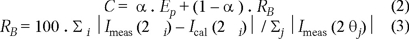The parameter α (0 ≤ α ≤ 1) is used to dose the contribution of each one of the parts. If the diffractogram has more relevance than the adopted model to describe the potential energy of the system, we can adopt a small value of α and thereby to increase the influence of the diffractometric data to the cost function. The basic hypothesis of the method is that the real structure of the compound corresponds to the minimum of this function.

The way of optimizing the cost function consists of generating initially an arbitrary model of structure that tries to respect some restrictive conditions such as minimum values to some relevant interatomic distances. The potential energy and the expected diffratogram corresponding to this structure are calculated and the initial value of cost function determined. Next, small arbitrary displacements are applied in the atoms and a new value of cost function is obtained. If this value is smaller than the previous one, this new structural configuration is accepted and it is used as the starting point to new displacements. If the value of the cost function is bigger than the initial value, then this new configuration is only accepted with a probability, a probability that is smaller, the bigger the difference is between the present cost function and the initial one. The probability is determined by the expression exp(- Δ C / K), where C is the variation of cost function and K is an arbitrary parameter. With this procedure it is possible, in the course of arbitrary displacements, to surpass local minima in the cost function —that has typically a very uneven topogra-phy with many local minima— and thus to converge to the absolute minimum.

This procedure is used in several optimization processes and it is called "simulating annealing". This nomenclature is chosen in analogy with the physical process of solidification, in which a liquid is converted to a crystalline state of lower energy by the gradual temperature lowering overcoming metastable states with more efficiency the lower the rate of cooling. Kirkpatrick  makes an interesting review of this method and its relation to mechanical statistics principles.

For the potential energy, Epot, we used a simple function involving the classic expression to a system of charges (qi . e) under Coulomb interaction added to an empirical repulsive term that sets limits to the minimum distances dijmin between the pair of ions (i, j),where e is the fundamental charge, ε0 is the permittivity of vacuum and k is a constant. The values of minimum distances can be selected from tabulated values according to the character of the expected bonds. The summation is evaluated by means of optimized Ewald's series [15, 17].

Results and discussion

The presented results on Table 2 were used as a starting point to locate the hydrogen with ENDEAVOUR. The model for the potential energy involves isolated ions and to the contribution of nitrogen of azide ion we attribute a fractional charge of -1/3 to each member of the group and a charge of -3 to the isolate nitrogen N(5). To hydrogen charges, +1 were attributed and their positions were left free for the optimization of the cost function with α = 1. The minimum distance N-H and H-H adopted for the repulsive term in Epot were 1.0 Å and 1.6 Å, respectively.

The optimization process converged to a tetrahedron arrangement around the nitrogen N(5) with two hydrogen, H(1) and H(2), in general positions of the group Pmna (Table 3). Each ammonium group consists of a pair H(1) and a pair H(2), internally related by a point symmetry 2 of the site N(5), with angles H-N-H that vary from 104.0° to 110.8° and distances N-H of about 1.0 Å.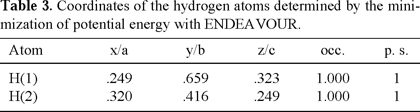The refinement of this structure model was performed, via Rietveld method, in two stages. First, we refined the nitrogen positions without the insertion of hydrogen in the asymmetric unit. The R-factors found were Rwp= 0.265 (Rexpected= 0.179) and S= 2.18. Secondly, we introduced the hydrogen atoms and refined their coordinate with constrains over the minimum distances N-H of 1.0 Å and H-H of 1.6 Å. The R-factors already presented a significant improvement in the first cycle of refinement and stabilized in values Rwp= 0.229 (Rexpected = 0.179) and S = 1.63. Isotropic thermal parameters were initially refined for each non-H atom. For H-atoms unreasonable values were obtained. At the final stage of refinement it was used a simplified description of thermal vibrations where we adopted one isotropic thermal parameters for each ion. The Fig. 1 presents the calculated diffratogram from the refinement results. On Table 4 we present the final results of the refinement and on Table 5 a list of the main interatomic distances in comparison with the values obtained by Prince and Choi  via single crystal neutron diffraction. In this last work, values are also given to the distances N-N in the azide group and N-H distances in the ammonium group, as refined from a rigid body model. In this approach the distances N(1)-N(2) and N(3)-N(4) are kept equal and the displacements of H(1) and H(2) are coupled through the riding model to the ammonium group . The average value of the distances to the two azide groups reported here is very close to the obtained value by Prince and Choi  with the rigid body approach.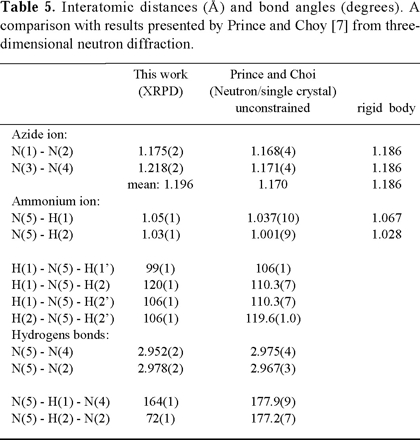The azide groups N(2)-N(1)-N(2) are aligned along the a-axis. The longitudinal axis of the azida groups N(4)-N(3)-N(4) are parallel to the plane (010) and form angles ('zig-zag' an-gle), between themselves, of about 139°. These crystallo-graphic differences between the two groups make the hypoth-esis of identical N-N distances somewhat artificial. The N(5) are donors and N(2) and N(4) acceptors of H-bonds. The va-lues of the angle N-H...N are characteristics of these bonds although a little smaller than values reported by Prince and Choi .

In the Fig. 3, we can observe the basic characteristics of the structure. The doted hydrogens (Fig. 2b) indicate the approximate positions with the minimization of potential ener-gy and the non-doted indicate the position reached at the refinement stage with the Rietveld method. The doted tetrahedron does not show an orientation that suits the presence of intense H-bonds with the nitrogen of azide groups as revealed by the previous neutron diffraction study. The angles which we obtained are N(5)-H(1)....N(2) ≅ 150 ° and N(5)-H(2)....N(4) ≅ 144 °. These results are fundamentally related to the adopted model of potential energy. The model contains only isotropic terms that are better adopted to strictly ionic compounds. With the structure refinement, these angles increase (Table 5) and become closer to the linear arrangement as indicated by the neutron diffraction.Although other models of charge distribution to the ions may be tried, still together with the Coulomb potential, the fact is that the starting data for hydrogen atoms were adequate enough for the structure refinement. This conclusion can be in fact more extended: even in a rather complex situation, a starting approximate structure model is sufficient to obtain convergence to the absolute minimum at the refinement stage with Rietveld method.

The probability of success in solving a crystal structure from x-ray powder diffraction data depends directly on the effective resolution of the experimental diffractogram. In the present case no cluster of severely superposed lines was observed, what put in evidence the tremendous advantages in the use of high-resolution x-ray powder diffractometer based on synchrotron light sources. Although it was not the purpose of this work we investigated the possibility to locate the hydrogen atoms directly via Fourier difference maps. The results presented in Table 2 were refined and the difference electron density synthesis, with coefficients ( | F(measured) | - | F(calculated) | ) and calculated phases (ϕ ), was constructed. Searching for peaks in the proximity of N(5), it was possible to locate the two hydrogen atoms, H(1) and H(2), in two general positions. The analysis was implemented with SHELXL-93 . The coordinates, of both atoms, are very close to those obtained by minimization of potential energy, listed in Table 3. Fig. 4 shows two sections of Fourier synthesis | F(measured) | . exp(- i.ϕ (calculated)): the planes containing H(1)-N(5)-H(1') and H(2)-N(5)-H(2'). We can observe the contributions of hydrogen atoms to scattered radiation. In fact, the complete structure of ammonium azide could be solved from powder data by the usual methodologies applied to monocrystal data. This possibility is, obviously, associated to the simplicity of the problem. In more complex structure, it becomes more evident the importance of introducing comple-mentary information beyond the x-ray, or neutron, diffraction context.Conclusions

The use of direct space strategies in connection to the princi-ple of minimization of potential energy seems to have a promising future as a powerful strategy to solve a crystal structure from powder data. Even to those cases, in which solving the structure completely from XRPD is impossible, many structural details could certainly be obtained.

The results presented here show that it was fully possible to reproduce ab initio, from XRPD, the essential results of the ammonium azide structure as previously determined from neutron scattering. In many situations, the level of structural detail reached here is more than sufficient to understand the chemical properties of the compound.

Acknowledgements

The authors thank FUJB, FAPERJ, FINEP and CNPq for their financial support. One of the authors (PP) would like to thank the Swiss National Science Foundation for financial support and for access to the Swiss-Norwegian Beamline. We are grateful to prof. W. Weyrich and Mr. R. Barreca in the Department of Chemistry of the University of Konstanz, Germany for kindly providing the sample used in this experiment.

Note: All the computer programs used in this work, as well as manuals and tutorials, can be obtained trough the web site http://www.ccp14.ac.uk. This is an excellent central site to obtain links and general information on crystallography and x-ray powder diffraction.

References

1. Poojary, D. M.; Cabeza, A ; Aranda, M. A. G; Bruque, S.; Clearfield, A. Inorg. Chem. 1996, 35, 1468 1473.         [ Links ]

2. Morris, R. E.; Owen, J. J.; Stalick, J. K.; Cheetham, A. K. J. Solid State Chem. 1994, 111, 52-57.         [ Links ]

3. Rietveld, H. M. J. Appl. Cryst 1969, 2, 65-71.         [ Links ]

4. Louër, D. Acta Cryst. 1998, A54, 922-933.         [ Links ]

5. Helliwell, J. R. Acta Cryst. 1998, A54, 738-749.         [ Links ]

6. Frevel, L. K. Z. Kristallogr. 1936, 94, 197-         [ Links ].

7. Prince, E.; Choi, C. S. Acta Cryst. 1978, B34, 2606-2608.         [ Links ]

8. Evans, L.; Yoffe, A. D.; Gray, P. Chemical Reviews (Washington, D.C.) 1959, 59, 515-568.         [ Links ]

9. Rodriguez-Carvajal, J. Abstracts of the Satellite Meeting on Powder Diffraction of the XV Congress of IUCr, Toulouse, France, 1990, p.127.         [ Links ]

10. Taupin, D. J. Appl. Cryst. 1973, 6, 380-385.         [ Links ]

11. Wolff, P. M. J. Appl. Cryst. 1968, 1, 108-113.         [ Links ]

12. Le Bail, A.; Duroy, H.; Fourquet, J. L. Mater. Res. Bull. 1988, 23, 447.         [ Links ]

13. Giacovazzo, C.; Monaco, H. L.; Viterbo, D.; Scordari, F.;Gilli, G.; Catti, M.: Fundamentals of Crystallography (IUCr Texts on Crystallography-2). Oxford University Press Inc., New York. 1995, 322.         [ Links ]

14. Altomare, A.; Burla, M. C.; Camalli, M.; Cascarano, G. L.; Giacovazzo, C.; Guagliardi, A.; Moliterne, A. G. G.; Polidori, G.; Spagna, R. J. Appl. Cryst. 1999, 32, 115-119.         [ Links ]

15. Putz, H.; Schön, J. C.; Jansen, M. J. Appl. Cryst. 1999, 32, 864-870.         [ Links ]

16. Kirkpatrick, S.; Gelatt Jr., C. D.; Vecchi, M. P. Science 1983, 220-232.         [ Links ]

17. Catti, M. Acta Cryst. 1978, A34, 974-979.         [ Links ]

18. Busing, W. R.; Levy, H. A. Acta Cryst. 1964, 17, 142-146.         [ Links ]

19. Sheldrick, G. M., Dauter, Z., Wilson, K. S., Hope, H.; Sieker, L. C. Acta Cryst. 1993, D49, 18-23.         [ Links ]

20. Young, R.A.: The Rietveld Method, IUCr - Oxford University Press, 1995.         [ Links ]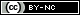Todo el contenido de esta revista, excepto dónde está identificado, está bajo una Licencia Creative Commons